# 用了 TS 映射类型，同事直呼内行！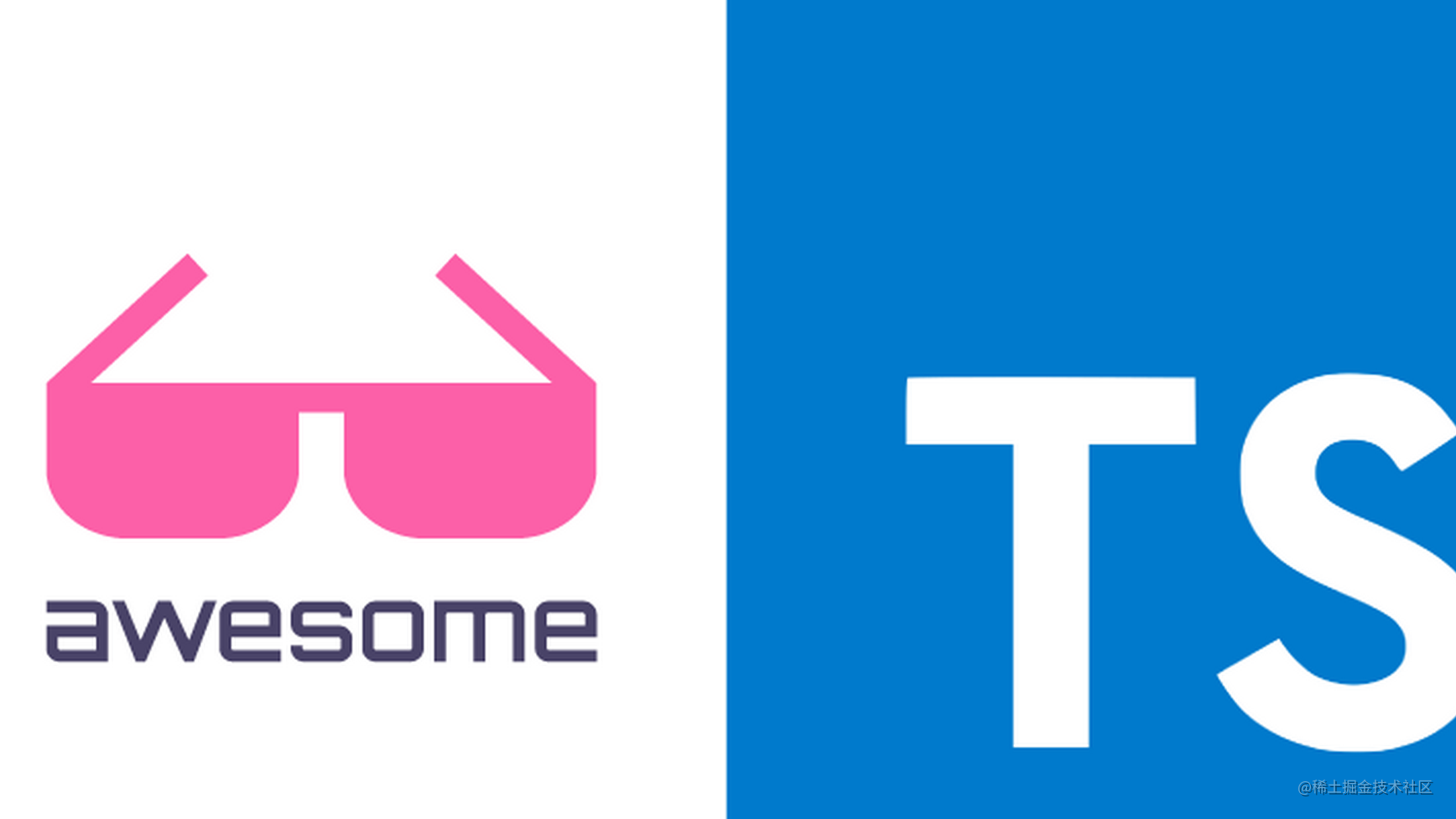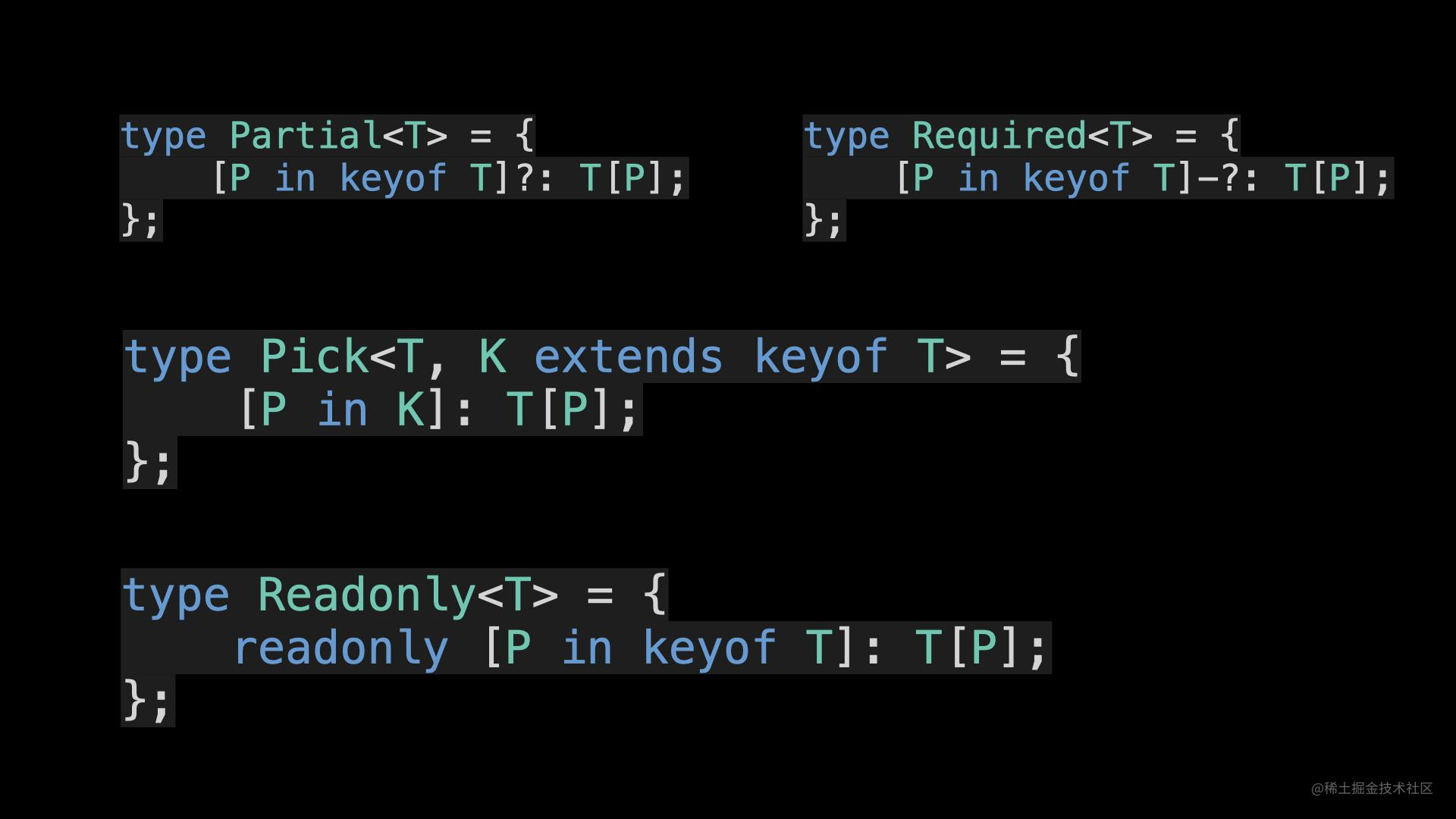type User = {
name: string; // 姓名
phone: string; // 联系电话
};

type UserPartial = {
name?: string; // 姓名
phone?: string; // 联系电话
};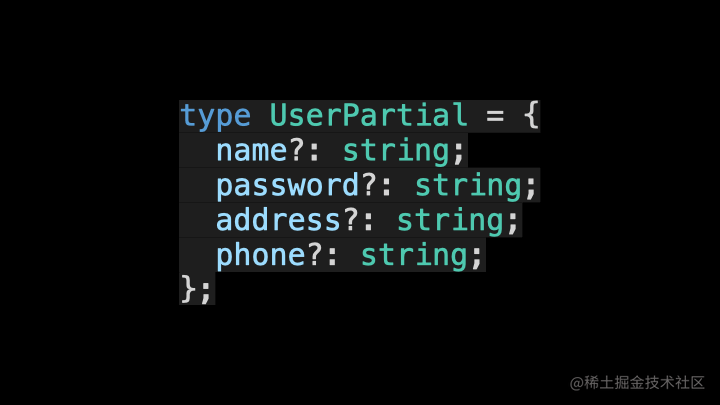type ReadonlyUser = {
};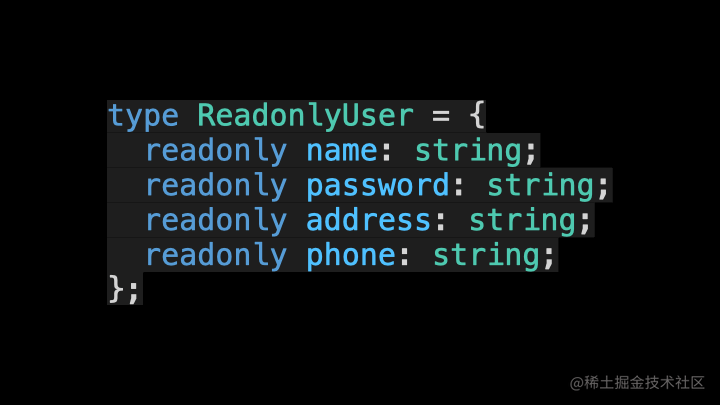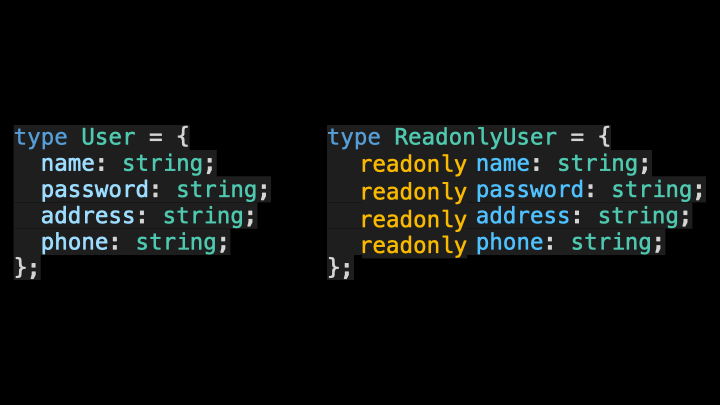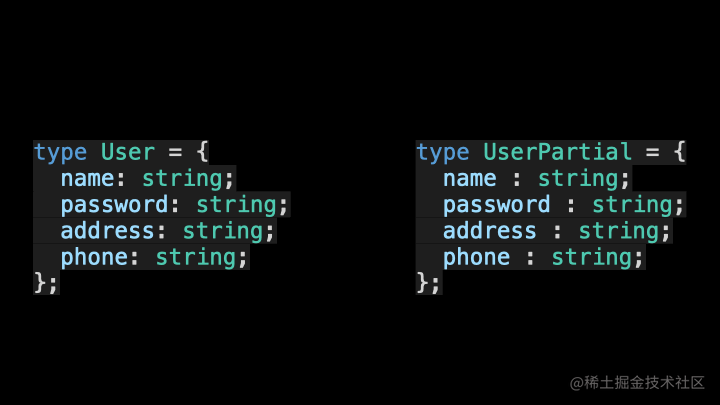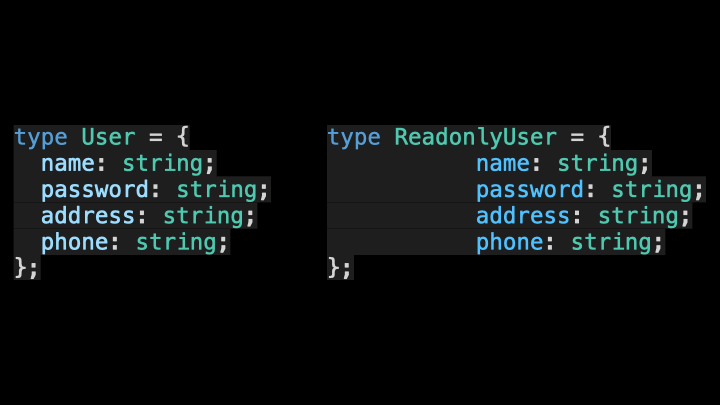{ [ P in K ] : T }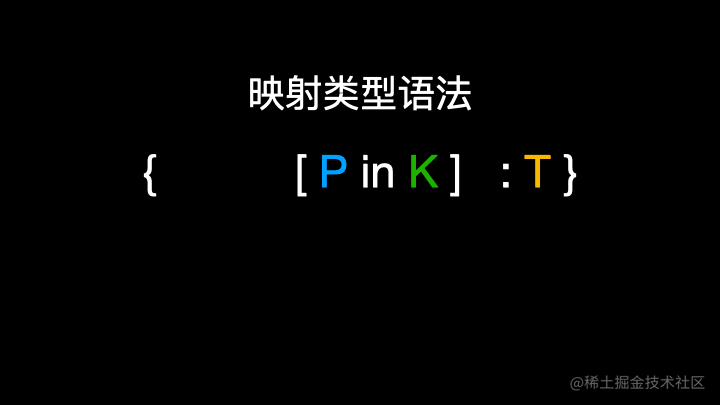{ [ P in K ] : T }
{ [ P in K ] ?: T }
{ [ P in K ] -?: T }
{ readonly [ P in K ] : T }
{ readonly [ P in K ] ?: T }
{ -readonly [ P in K ] ?: T }

type Item = { a: string; b: number; c: boolean };

type T1 = { [P in "x" | "y"]: number }; // { x: number, y: number }
type T2 = { [P in "x" | "y"]: P }; // { x: "x", y: "y" }
type T3 = { [P in "a" | "b"]: Item[P] }; // { a: string, b: number }
type T4 = { [P in keyof Item]: Item[P] }; // { a: string, b: number, c: boolean }

type MyPartial<T> = {
[P in keyof T]?: T[P];
};

type UserPartial = MyPartial<User>;TypeScript 4.1 版本允许我们使用 as 子句对映射类型中的键进行重新映射。它的语法如下：

type MappedTypeWithNewKeys<T> = {
[K in keyof T as NewKeyType]: T[K]
//            ^^^^^^^^^^^^^
//            这是新的语法！
}type Getters<T> = {
[K in keyof T as get\${Capitalize<string & K>}]: () => T[K]
};

interface Person {
name: string;
age: number;
location: string;
}

type LazyPerson = Getters<Person>;
// {
//   getName: () => string;
//   getAge: () => number;
//   getLocation: () => string;
// }// Remove the 'kind' property
type RemoveKindField<T> = {
[K in keyof T as Exclude<K, "kind">]: T[K]
};

interface Circle {
kind: "circle";
}

type KindlessCircle = RemoveKindField<Circle>;
//   type KindlessCircle = {
//   };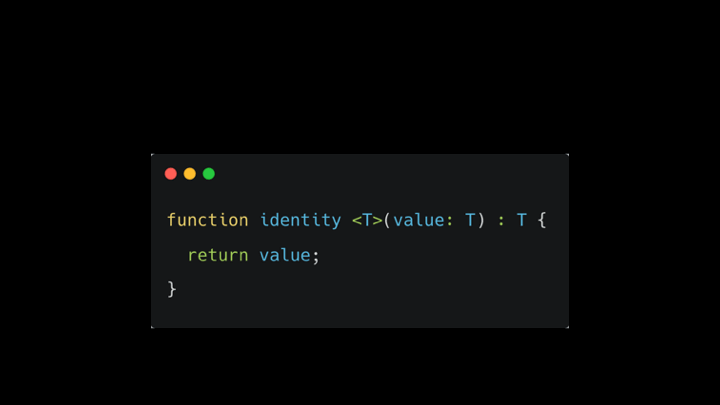TypeScript 泛型中的 K、T、V 等到底是个啥？